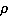### Create an Account

Home / Questions / A cylindrical buoy with a radius of 15 m and a mass of 1000 kg floats in salt water 1026 ...

# A cylindrical buoy with a radius of 15 m and a mass of 1000 kg floats in salt water 1026 kgm3 Determine the natural frequency of this system 2 The static displacement of a system with a

A cylindrical buoy with a radius of 1.5 m and a mass of 1000 kg floats in salt water (= 1026 kg/m3). Determine the natural frequency of this system.

2. The static displacement of a system with a motor weight of 385.6 kg is found to be 0.0254 mm. Determine the natural frequency of vertical vibrations of this system.

Jun 25 2020 View more View LessSubscribe To Get Solution